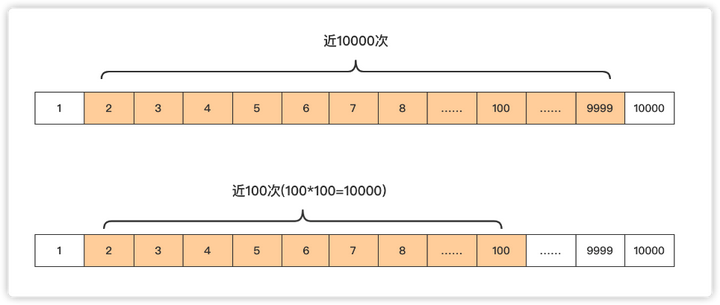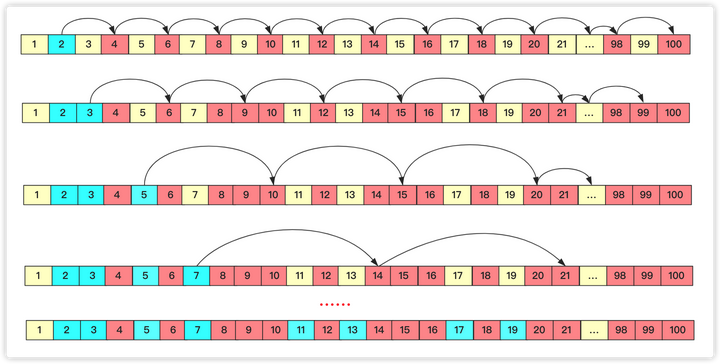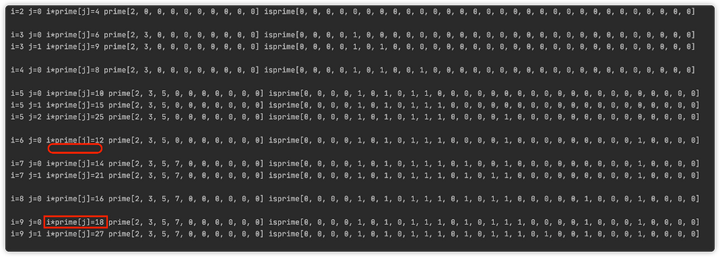# 面试官：你知道怎么求素数吗？

2021/06/28 14:28

## 求一个质数

boolean isprime(int value){
for(int i=2;i<value;i++)
{
if(value%i==0)
{return false;}
}
return true;
}

boolean isprime(int value)
{
for(int i=2;i*i<value+1;i++)
{
if(value%i==0)
{return false;}
}
return true;
}## 求多个素数

### 埃拉托斯特尼(Eratosthenes)筛法boolean isprime[];
long prime[];
void getprime()
{
prime=new long;//记录第几个prime
int index=0;//标记prime当前下标
isprime=new boolean ;//判断是否被标记过
for(int i=2;i<1000001;i++)
{
if(!isprime[i])
{
prime[index++]=i;
}
for(int j=i+i;j<1000000;j=j+i)//他的所有倍数都over
{
isprime[j]=true;
}
}
}

### 欧拉筛

• 在遍历到一个数的时候，如果这个数没被标记，那么这个数存在素数的数组中，对应下标加1.
• 不管这个数是不是素数，遍历已知素数将它和该素数的乘积值标记，如果这个素数能够被当前值i整除，那么停止操作进行下一轮。

boolean isprime[];
int prime[];
void getprimeoula()// 欧拉筛
{
prime = new int;// 记录第几个prime
int index = 0;
isprime = new boolean;
for (int i = 2; i < 1000001; i++) {
if (!isprime[i]) {
prime[index++] = i;
}
for (int j = 0; j < index && i * prime[j] <= 100000; j++){//已知素数范围内枚举
isprime[i * prime[j]] = true;// 标记乘积
if (i % prime[j] == 0)
break;
}
}
}

i*prime[j+1]=(prime[j]*k)*prime[j+1]=prime[j]*(k*prime[j+1]) 当i=k*prime[j+1]两个位置就产生冲突重复计算啦,所以一旦遇到能够被整除的就停止。0 评论
0 收藏
0